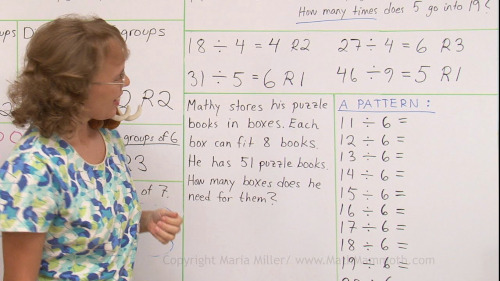^

# Math videos for division, long division, factors, divisibility, and primes (grades 3-5)

Find here free online math videos on division, long division, factors, divisibility, and primes, meant for 3rd, 4th, and 5th grades.

## Division with single-digit divisor (3rd grade)

These videos match topics in Math Mammoth Division 1 worktext (Blue Series).

The connection between multiplication and division

Divide evenly into groups — basic concept of division

Division versus multiplication word problems

Division by zero

Division with remainders

These videos match topics in Math Mammoth Division 2 worktext (Blue Series).The order of operations plus writing simple expressions

The remainder, part 1

The remainder, part 2 – we learn to write division with a different symbol and learn about the remainder in word problems

How to teach long division

Long division — step-by-step examples (several videos)

Remainder problems – word problems involving long division with a remainder

Concept of average

Find fractional parts with division

These videos match topics in Math Mammoth Multiplication & Division 3 worktext (Blue Series).

Review: Multiplication and Division – simple equations, bar models, terminology

Long division with a two-digit divisor

Long division as continued (repeated) subtraction

## Divisibility, factors, and primes (grades 4-6)

These videos match the topics in Math Mammoth Factors & Factoring worktext (Blue Series).

Divisibility (and factors & multiples)

Divisibility rules

An introduction to prime numbers

Finding factors

Prime factorization using factor trees

The sieve of Eratosthenes (for finding primes)

Prime factorization

The greatest common factor

The least common multiple

How to use factoring to simplify fractions, and how to cross cancel

WAIT!

Receive my monthly collection of math tips & resources directly in your inbox — and get a FREE Math Mammoth book!You can unsubscribe at any time.

### Math Mammoth Tour

Confused about the different options? Take a virtual email tour around Math Mammoth! You'll receive:

An initial email to download your GIFT of over 400 free worksheets and sample pages from my books. Six other "TOURSTOP" emails that explain the important things and commonly asked questions concerning Math Mammoth curriculum. (Find out the differences between all these different-colored series!)

This way, you'll have time to digest the information over one or two weeks, plus an opportunity to ask me personally about the curriculum.
A monthly collection of math teaching tips & Math Mammoth updates (unsubscribe any time)### "Mini" Math Teaching Course

This is a little "virtual" 2-week course, where you will receive emails on important topics on teaching math, including:

- How to help a student who is behind
- Troubles with word problems
- Teaching multiplication tables
- Why fractions are so difficult
- The value of mistakes
- Should you use timed tests
- And more!

A GIFT of over 400 free worksheets and sample pages from my books right in the very beginning.A monthly collection of math teaching tips & Math Mammoth updates (unsubscribe any time)
Enter your email to receive math teaching tips, resources, Math Mammoth news & sales, humor, and more! I tend to send out these tips about once monthly, near the beginning of the month, but occasionally you may hear from me twice per month (and sometimes less often).• A GIFT of over 400 free worksheets and sample pages from my books.